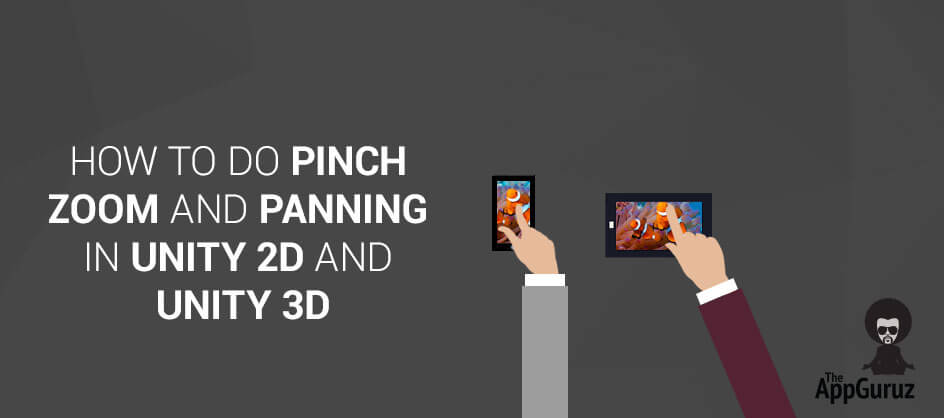## Objective

The main objective of this code sample is to explain how to implement pinch zoom and panning functionality in Unity2D and Unity3D.

## Step 1 Introduction

In this sample code, the pinch zoom and panning functionality have been implemented. It has same behavior like when we zoom and pan/drag image in our phone gallery. This sample code will work on both unity2d and unity3d objects. It’s independent from camera; no matter either main camera is tk2dcamera or simple Camera and its projection type is orthographic or perspective.

## Step 2 Example

The following simple c# code will do zoom in and zoom out from the position where you pinch through fingers and pan the image on single touch move.

### Note

Add following script on GameObject on which you want to implement Pinch Zoom functionality.

### 2.1 PinchZoomScript

``````using UnityEngine;
using System.Collections;

public class PinchZoom : MonoBehaviour
{
private float scale_factor= 0.07f;
private float MAXSCALE = 6.0f, MIN_SCALE = 0.6f; // zoom-in and zoom-out limits
private bool isMousePressed;
private Vector2 prevDist = new Vector2(0,0);
private Vector2 curDist = new Vector2(0,0);
private Vector2 midPoint = new Vector2(0,0);
private Vector2 ScreenSize;
private Vector3 originalPos;
private GameObject parentObject;

void Start ()
{
// Game Object will be created and make current object as its child (only because we can set virtual anchor point of gameobject and can zoom in and zoom out from particular position)
parentObject = new GameObject("ParentObject");
parentObject.transform.parent = transform.parent;
parentObject.transform.position = new Vector3(transform.position.x*-1, transform.position.y*-1, transform.position.z);
transform.parent = parentObject.transform;

ScreenSize = Camera.main.ScreenToWorldPoint(new Vector2(Screen.width,Screen.height));
originalPos = transform.position;
isMousePressed = false;
}
void Update ()
{
if(Input.GetMouseButtonDown(0))
isMousePressed = true;
else if(Input.GetMouseButtonUp(0))
isMousePressed = false;
// These lines of code will pan/drag the object around untill the edge of the image
if(isMousePressed && Input.touchCount==1 && Input.GetTouch(0).phase == TouchPhase.Moved && (parentObject.transform.localScale.x > MIN_SCALE || parentObject.transform.localScale.y > MIN_SCALE))
{
Touch touch = Input.GetTouch(0);
Vector3 diff = touch.deltaPosition*0.1f;
Vector3 pos = transform.position + diff;
if(pos.x > ScreenSize.x * (parentObject.transform.localScale.x-1))
pos.x = ScreenSize.x * (parentObject.transform.localScale.x-1);
if(pos.x < ScreenSize.x * (parentObject.transform.localScale.x-1)*-1)
pos.x = ScreenSize.x * (parentObject.transform.localScale.x-1)*-1;
if(pos.y > ScreenSize.y * (parentObject.transform.localScale.y-1))
pos.y = ScreenSize.y * (parentObject.transform.localScale.y-1);
if(pos.y < ScreenSize.y * (parentObject.transform.localScale.y-1)*-1)
pos.y = ScreenSize.y * (parentObject.transform.localScale.y-1)*-1;
transform.position = pos;
}
// On double tap image will be set at original position and scale
else if(Input.touchCount==1 && Input.GetTouch(0).phase == TouchPhase.Began && Input.GetTouch(0).tapCount==2)
{
parentObject.transform.localScale = Vector3.one;
parentObject.transform.position = new Vector3(originalPos.x*-1, originalPos.y*-1, originalPos.z);
transform.position = originalPos;
}
checkForMultiTouch();
}
// Following method check multi touch
private void checkForMultiTouch()
{
// These lines of code will take the distance between two touches and zoom in - zoom out at middle point between them
if (Input.touchCount == 2 && Input.GetTouch(0).phase == TouchPhase.Moved && Input.GetTouch(1).phase == TouchPhase.Moved)
{
midPoint = new Vector2(((Input.GetTouch(0).position.x + Input.GetTouch(1).position.x)/2), ((Input.GetTouch(0).position.y + Input.GetTouch(1).position.y)/2));
midPoint = Camera.main.ScreenToWorldPoint(midPoint);

curDist = Input.GetTouch(0).position - Input.GetTouch(1).position; //current distance between finger touches
prevDist = ((Input.GetTouch(0).position - Input.GetTouch(0).deltaPosition) - (Input.GetTouch(1).position - Input.GetTouch(1).deltaPosition)); //difference in previous locations using delta positions
float touchDelta = curDist.magnitude - prevDist.magnitude;
// Zoom out
if(touchDelta>0)
{
if(parentObject.transform.localScale.x < MAXSCALE && parentObject.transform.localScale.y < MAXSCALE)
{
Vector3 scale = new Vector3(parentObject.transform.localScale.x + scale_factor, parentObject.transform.localScale.y + scale_factor, 1);
scale.x = (scale.x > MAXSCALE) ? MAXSCALE : scale.x;
scale.y = (scale.y > MAXSCALE) ? MAXSCALE : scale.y;
scaleFromPosition(scale,midPoint);
}
}
//Zoom in
else if(touchDelta<0)
{
if(parentObject.transform.localScale.x > MIN_SCALE && parentObject.transform.localScale.y > MIN_SCALE)
{
Vector3 scale = new Vector3(parentObject.transform.localScale.x + scale_factor*-1, parentObject.transform.localScale.y + scale_factor*-1, 1);
scale.x = (scale.x < MIN_SCALE) ? MIN_SCALE : scale.x;
scale.y = (scale.y < MIN_SCALE) ? MIN_SCALE : scale.y;
scaleFromPosition(scale,midPoint);
}
}
}
// On touch end just check whether image is within screen or not
else if (Input.touchCount == 2 && (Input.GetTouch(0).phase == TouchPhase.Ended || Input.GetTouch(0).phase == TouchPhase.Canceled || Input.GetTouch(1).phase == TouchPhase.Ended || Input.GetTouch(1).phase == TouchPhase.Canceled))
{
if(parentObject.transform.localScale.x < 1 || parentObject.transform.localScale.y < 1)
{
parentObject.transform.localScale = Vector3.one;
parentObject.transform.position = new Vector3(originalPos.x*-1, originalPos.y*-1, originalPos.z);
transform.position = originalPos;
}
else
{
Vector3 pos = transform.position;
if(pos.x > ScreenSize.x * (parentObject.transform.localScale.x-1))
pos.x = ScreenSize.x * (parentObject.transform.localScale.x-1);
if(pos.x < ScreenSize.x * (parentObject.transform.localScale.x-1)*-1)
pos.x = ScreenSize.x * (parentObject.transform.localScale.x-1)*-1;
if(pos.y > ScreenSize.y * (parentObject.transform.localScale.y-1))
pos.y = ScreenSize.y * (parentObject.transform.localScale.y-1);
if(pos.y < ScreenSize.y * (parentObject.transform.localScale.y-1)*-1)
pos.y = ScreenSize.y * (parentObject.transform.localScale.y-1)*-1;
transform.position = pos;
}
}
}
//Following method scales the gameobject from particular position
static Vector3 prevPos = Vector3.zero;
private void scaleFromPosition(Vector3 scale, Vector3 fromPos)
{
if(!fromPos.Equals(prevPos))
{
Vector3 prevParentPos = parentObject.transform.position;
parentObject.transform.position = fromPos;
Vector3 diff = parentObject.transform.position - prevParentPos;
Vector3 pos = new Vector3(diff.x/parentObject.transform.localScale.x*-1, diff.y/parentObject.transform.localScale.y*-1, transform.position.z);
transform.localPosition = new Vector3(transform.localPosition.x + pos.x, transform.localPosition.y+pos.y, pos.z);
}
parentObject.transform.localScale = scale;
prevPos = fromPos;
}
}``````

If you have got any query related to How to do Pinch zoom and panning in unity? please mention it in comment we will try to solve it out.

Got an Idea of Game Development? What are you still waiting for? Contact us now and see the Idea live soon. Our company has been named as one of the best Game Development Company in India.I am professional Game Developer, developing games in cocos2d(for iOS) and unity(for all platforms). Games are my passion and I aim to create addictive, high quality games. I have been working in this field since 1 year.